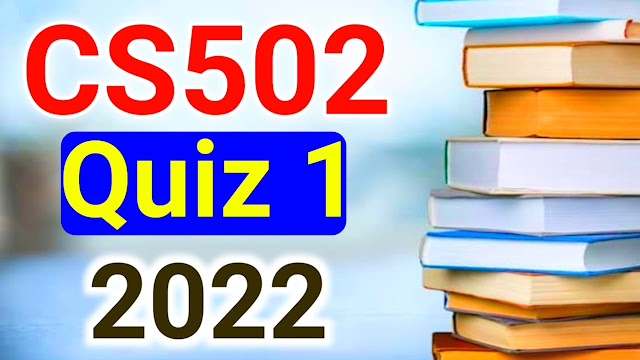# CS502 Quiz 1 Solution

### CS502 - Fundamentals of Algorithms MCQs

 Post Title CS502 Quiz 1 Solution 2022 Book Code CS502 Degree BSIT/BSCS/Other University Virtual Univerity of Pakistan CS502 Quiz 1/ Quiz 2 / Quiz 3 CS502 Quiz 1 Mid/Final CS502 Quiz 1 Midterm MCQs Also Read CS502 Quiz 1 Solved Also Read CS502 Lecture Wise MCQs For Midterm

## CS502 Quiz 1 Solution

There are no hard formal rules to the syntax of the …………code.  (CS502)

Pseudo

Programming

Assembly

Basic

………..provides us more accurate results, when input values are not closer with each other.  (CS502)

Mode

Average

Median

Mean

To find maximal points in a brute-force algorithm each point at the space is compared against…………of that space.  (CS502)

Most of the other points

All other points

One other point

Few other points

In addition to passing in the array itself to merge sort algorithms we will pass in ………..other arguments which are indices.  (CS502)

3

2

5

4

If the time complexity of an algorithm is given by O (1), then its time complexity would be.  (CS502)

Polynomial

Constant

Exponential

Average

Which one of the following is the worst-case time complexity of merge sort?  (CS502)

O(nlogn)

O[(n^2logn]

O[nlogn^2]

O[n^2]

The words of algebra and algorithm were derived from?  (CS502)

Title of Al-Khwarizmi writings and his name

Al-Khwarizmi writings

Al-Khwarizmi name

Book of algebra

The approach of solving geometric problems by sweeping a line across the plane is called ………..sweep.  (CS502)

Cube

Line

Plane

Box

Using ………….algorithm, efficiency is not given much importance.  (CS502)

Processing

Brute force

Merge sort

Greedy

In the following code the statement cout <<j  executes …………times. For (int i = 1, i< =n;i++) cout<<i;  (CS502)

Infinite times

N+5 times

Zero times

N times

In addition to passing in the array itself to merge sort algorithms, we will pass in ………..other arguments which are incidents.  (CS502)

5

2

4

3

The process of …….ends when you are left with such tiny pieces remaining that it is trivial t-o solve them.  (CS502)

Brute - force

Axis - sweep

Divide and conquer

Plan sweep

The total no of arguments passed to merge sort algorithm is ……………….(CS502)

4

3

5

2

In  2d maxima problem, a point is said to be ……….if it is not dominated by any other point in that space.   (CS502)

Maximal

Minimal

Member

Joint

A number of ………….of the pseudo code are counted to measure the running time.  (CS502)

Pages

Outputs

Inputs

Steps

While analysis of the brute-force maxima algorithm, on array sorted in the reverse order is the type of ……….code input.  (CS502)

Average

BestCS502 Quiz 1 Solution 2022